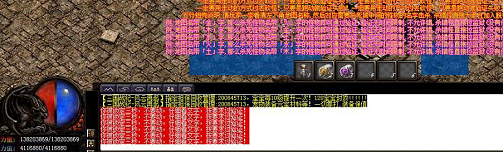# 七星辅助过只能走动打金木水火土防脱机验证挂机脚本这个防脱机验证就是根据 提示打指定的怪，但是怪物的位置基本不变，思路就是先走到需要打的怪附近，然后获取指定名字的怪，获取到之后走到怪物附近，然后进行攻击。这个脚本在七星辅助更新了参数之后就变得很容易了。

七星辅助官网为大家介绍过一种大金木水火土怪的防脱机验证，但是现在又新出了一种类似的防脱机验证，只不过检测的更加严格了，进去地图之后只能走路，跑动的话就会被锁定三秒。大家还记得之前分享过的七星辅助B版禁止跑的参数没有，现在正好派上用场，一起来看下。

<土怪物>
/<

goods = 获取环境信息(1,"*土守卫")
for k,v in pairs(goods) do  -- 遍历数组
print(v.x,v.y)  -- 打印X坐标
if v.x and v.y then
走到(v.x,v.y+1)

end
end

/>

<火怪物>

/<

goods = 获取环境信息(1,"*火守卫")
for k,v in pairs(goods) do  -- 遍历数组
print(v.x,v.y)  -- 打印X坐标
if v.x and v.y then
走到(v.x,v.y+1)

end
end

/>

<金怪物>

/<

goods = 获取环境信息(1,"*金守卫")
for k,v in pairs(goods) do  -- 遍历数组
print(v.x,v.y)  -- 打印X坐标
if v.x and v.y then
走到(v.x,v.y+1)

end
end

/>

<水怪物>

/<

goods = 获取环境信息(1,"*水守卫")
for k,v in pairs(goods) do  -- 遍历数组
print(v.x,v.y)  -- 打印X坐标
if v.x and v.y then
走到(v.x,v.y+1)

end
end

/>

<木怪物>

/<

goods = 获取环境信息(1,"*木守卫")
for k,v in pairs(goods) do  -- 遍历数组
print(v.x,v.y)  -- 打印X坐标
if v.x and v.y then
走到(v.x,v.y+1)

end
end

/>

从代码可以看出来就是走到怪物旁边，然后攻击指定怪物。这个防脱机验证码唯一的难度就是全程不能跑步，一定要保持走路状态。如果您还有不懂的地方可以直接咨询七星辅助官网客服，这样方便大家更好的使用七星辅助。

85%的户主都看了以下辅助挂机脚本下载